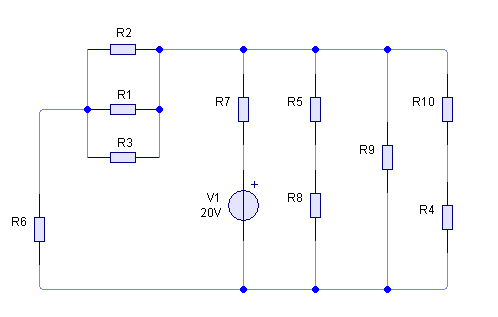# Resistance series and parallel

• bathtubbuddy

## Homework Statement## Homework Equations

series - Ra+Rb

parallel - 1/[(1/Ra)+(1/Rb)]

## The Attempt at a Solution

Im positive that R1,R2 y R3 are parallel, but also (R5+R8), R9 and (R10+R4) right? is R7 parallel to anything? which ones would be series? so confusing... thankies :]

bathtubbuddy said:
Im positive that R1,R2 y R3 are parallel,
Yes
but also (R5+R8), R9 and (R10+R4) right?
These 3 branches are parallel branches.
is R7 parallel to anything?
R7 is not. It is in series with a voltage source.
which ones would be series? so confusing..
Two branches are in series if [all of] the current that flows through one also then flows through the other.